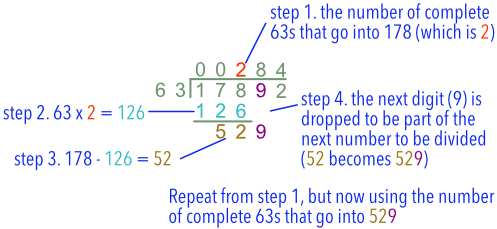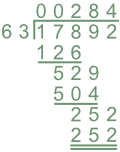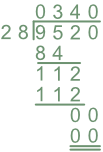Long Division

# Long Division

Basic, GCSE(F),

Long Division is used for larger numbers. It consists of a repeated sequence of four steps:

• Determine the how many times a number can be divided;
• Multiply the divisor by that multiple;
• Set out the remainder;
• Drop the next digit down to create a new number.

Division may not always give you an exact answer. Show any remainder as part of the answer.## Examples

1. Divide 17892 by 63.

Set out the division as shown below, with the divisor on the left on the left of the bus stop.Working from left to right, 63 does not go into the first number (1) or the first two numbers (17). The first number that can be divided is 178.

63 divides into 178 twice: place 2 in the answer line (above the 8). Put the result of 2 x 63 (=126) below the 178.  Draw a line below the 126.

Subtract 126 from 178 to obtain a remainder, 52.  Drop the next digit, 9, down to make a new number from the remainder and the dropped number: 529:

Repeat the steps again until the number has been completely divided.

2. There are 9520 bottles to be sent from a drinks warehouse to a wholesaler.  The bottles are put into boxes.  Each box contains 28 bottles. How many boxes will be required for the shipment?